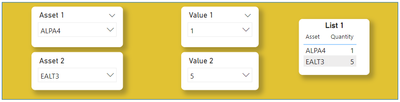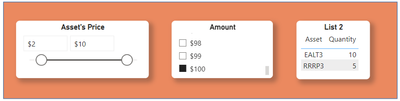cancel
Showing results for
Did you mean:Resolver I

## How to interact the results of two independent tables

Hi folks!
Does anyone could give me some advice on this problem please? Special thanks to @v-jingzhang, for helping me out with point 1 of this question!

I have two independent tables, and would like to do their results interact. As data, I have some Asset Codes and its Prices and Amount (\$ to buy the assets).

The context is:

1) I have "List 1", that is a table in which you select which Asset Code you own, and how many of each one, and the list aggregates these selections*tables used in this step: 'Asset Code 1', 'Asset Code 2', 'Value 1', 'Value 2' and 'Dim Asset'.

2) I have "List 2", that is a table filtered by the slicers "Asset's Price" and "Amount".
'Amount' is a series of numbers from \$1 to \$100, and is used also in measures to determine the quantities bought of each asset, like this: after I choose the range that I want to set at the price slicer (for ex. I want assets that have prices between "\$2 and \$10"), I'm left with RRRP3 (price = \$10) and EALT3 (price = \$5). Supposing that I choose \$100 at the "amount" slicer, and since I have two asset codes (thus \$50 for each), RRRP3 will have a quantity of 5 units and EALT3 will have 10 units.*tables used in this step: 'Asset Code 3' and 'Amount'.

3) I'm trying to create "Goal List", which is a table that is the subtraction of the quantities of List 1 from List 2, like this:

List 1                               List 2                        Goal List

Asset    Quantity             Asset    Quantity          Asset    Quantity

ALPA4        1                   RRRP3       5                 ALPA4        -1

EALT3         5                   EALT3       10               RRRP3         5

EALT3          5

ALPA would be equal to -1 because I don't have it anymore on my list (so it indicates that I have to sell the one that I have), as well as EALT3 equals 5 because it means that I would have to buy 5 more units of it.

Do you know if/how it would be possible?

Thank you very much 🙏

1 ACCEPTED SOLUTIONCommunity Support

Hi @LucaixBolha ,

I suggest you to try this measure.

``````Measure =
VAR _SUMMARIZE = SUMMARIZE('Dim Asset','Dim Asset'[Asset],"List 1",[Quantity],"List 2",CALCULATE([Quantity Asset Code 3],FILTER('Asset Code 3','Asset Code 3'[Asset] =EARLIER([Asset])))/2)
RETURN

Result is as below.Best Regards,
Rico Zhou

If this post helps, then please consider Accept it as the solution to help the other members find it more quickly.

4 REPLIES 4Super User

Wouldn't it be easier to UNION these tables, one with positive sign and the other with negative sign ?Resolver I

Thank you very much for your answer @lbendlin, I'll try it out!Community Support

Hi @LucaixBolha ,

I suggest you to try this measure.

``````Measure =
VAR _SUMMARIZE = SUMMARIZE('Dim Asset','Dim Asset'[Asset],"List 1",[Quantity],"List 2",CALCULATE([Quantity Asset Code 3],FILTER('Asset Code 3','Asset Code 3'[Asset] =EARLIER([Asset])))/2)
RETURN

Result is as below.Best Regards,
Rico Zhou

If this post helps, then please consider Accept it as the solution to help the other members find it more quickly.Resolver I

Thank you very much @v-rzhou-msft! It was exactly this that I was looking for 😄Announcements#### Exclusive opportunity for Women!

Join us for a free, hands-on Microsoft workshop led by women trainers for women where you will learn how to build a Dashboard in a Day!#### Power Platform Conference-Power BI and Fabric Sessions

Join us Oct 1 - 6 in Las Vegas for the Microsoft Power Platform Conference.Top Solution Authors
Top Kudoed Authors
Users online (2,358)